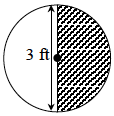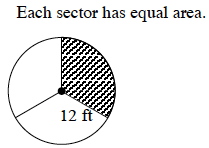### Home > MC2 > Chapter 8 > Lesson 8.1.1 > Problem8-10

8-10.

Find the area of each shaded sector (region) in the circles below. Write an exact answer in terms of $π$ and an approximate answer as a decimal.

Find the area of the shaded region based on the portion of the circle that it represents multiplied by the area of the total circle.
Area of a circle $= π · r^2$

1.$1.125 ≈ 3.53$ square feet

1.The shaded portion from part (b) is a third of the entire circle.